Courses
Courses for Kids
Free study material
Offline Centres
MoreLast updated date: 05th Dec 2023
Total views: 98.1k
Views today: 1.98kWhen we see a tabletop or a handkerchief, the basic quadrilateral shapes—a rectangle or a square—come to mind. There are many numerous varieties of quadrilaterals, and the shapes of the quadrilaterals themselves can recognize the types of quadrilaterals. The word is made up of two Latin words, Quadri, which is a variation of four, and latus, which signifies sides, as suggested by the name. A quadrilateral is a two-dimensional polygon with four sides. Quadrilaterals include the following 2D shapes: square, rectangle, rhombus, trapezium, parallelogram, and kite.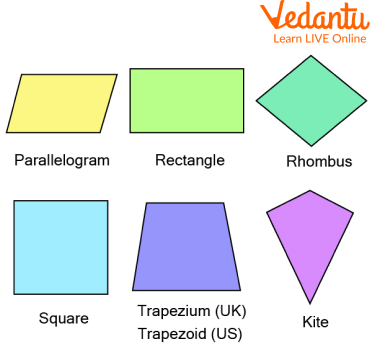A quadrilateral is a form of a polygon that consists of four sides, four vertices, four angles, and two diagonals.

The properties of a quadrilateral set it apart from a normal polygon in various ways. We have listed some common properties of quadrilaterals below:

• They must have four sides.

• They must have four vertices.

• They must have two diagonals.

• 360° is the total sum of interior angles.

There are various types of quadrilaterals, depending on different characteristics, and unique shapes. Just by looking at the quadrilateral shapes, one can tell the differences and qualities between them. Let's briefly discuss some of the types of quadrilaterals in this section.

Square: A quadrilateral with four equal sides and angles is called a square. The fact is that its sides and angles are equal, making it a regular quadrilateral. A square has four 90° angles. It can alternatively be viewed as a rectangle with equal lengths on its two neighbouring edges.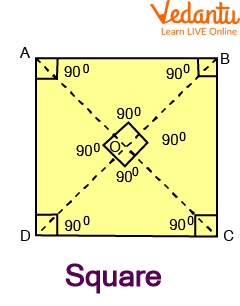Square

Sides: $A B=C D$ and $B C=A D$

Diagonals: $\mathrm{AO}=\mathrm{OC}$ and $\mathrm{DO}=\mathrm{OB}$

Angles: $\angle A=\angle C$ and $\angle B=\angle D=90^{\circ}$

If a square's side is "a," then

The square's area is equal to $a\times a =a^{2}$

The Square's perimeter is equal to 4a.

Rectangle: A rectangle contains four corners and four sides where opposite sides are of the same length and parallel to each other. The angles of a rectangle are equal in measure and are right-angled i.e. they measure $90^{\circ}$.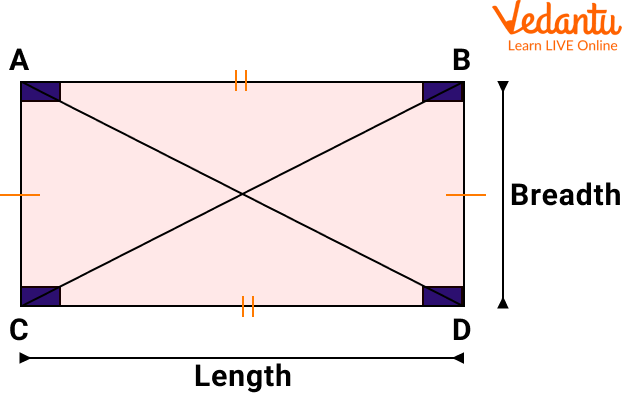Rectangle

## Some of the properties of the rectangle are given below:

• Two pairs of parallel sides.

• All four angles are right angles, that is, they measure 90 degrees.

• Opposite sides are of equal lengths.

• Two equal diagonals

In a rectangle, the two diagonals bisect each other in equal halves In the rectangle $P Q R S, P Q\|R S, P Q=R S, P S\| Q R$, and $P S=Q R$. All the angles are $90^{\circ}$ angles.

Kite: A kite has various names such as a dart or an arrowhead because of the shape. A kite has two pairs of equal-length sides and these sides are adjacent to each other.

Some of the properties of the kite are given below:

• Contains four edges and four vertices.

• Contains one line of symmetry.

• Contains two pairs of congruent and consecutive sides.

• Diagonals are perpendicular to each other.

In the kite $P Q R S, P Q=Q R$, and $P S=S R$.Kite

Parallelogram: As the name implies, a parallelogram is a simple quadrilateral with opposite sides as parallel. It, therefore, has two sets of parallel sides. A parallelogram also has its opposite angles as equal. In addition, the diagonals of a parallelogram cut each other. The sum of any two adjacent angles equals 180°.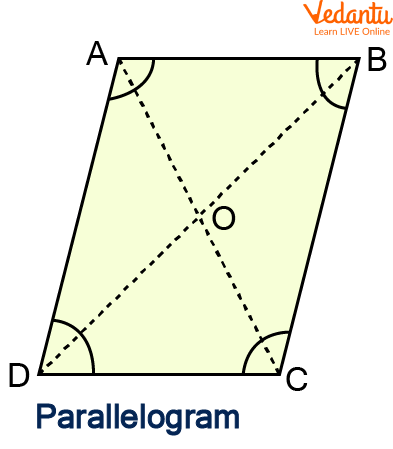Parallelogram

Sides: $A B=C D$ and $B C=A D$

Diagonals: $\mathrm{AO}=\mathrm{OC}$ and $\mathrm{DO}=\mathrm{OB}$

Angles: $\angle A=\angle C$ and $\angle B=\angle D$

If a parallelogram's length is I, breadth is $b$, and height is $h$, then

Parallelogram's perimeter equals $2 \times(\mathrm{l}+\mathrm{b})$.

Parallelogram's area equals $\mathrm{l} \times \mathrm{h}$

Rhombus: A quadrilateral called rhombus has four equal sides that are non-parallel to one another. The angles are not exactly 90 degrees. Right angles transform a rhombus into a square. In recognition of its similarities to the diamond card of playing cards, the rhombus is sometimes known as the "diamond."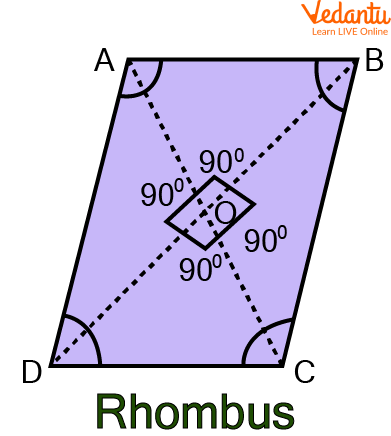Rhombus

Sides: $A B=C D$ and $B C=A D$

Diagonals: $\mathrm{AO}=\mathrm{OC}$ and $\mathrm{DO}=\mathrm{OB}$

Angles: $\angle A=\angle C$ and $\angle B=\angle D$

A rhombus's perimeter is equal to 4a if its side is a.

If two of the rhombus' diagonals are $d_{1}$ and $d_{2}$, then the area of a rhombus is equal to $\dfrac{1}{2} \times d_{1} \times d_{2}$

Trapezium: One pair of parallel sides make up a quadrilateral known as a trapezium. The two other sides are referred to as "legs" or "lateral sides," whereas the parallel sides are known as "bases."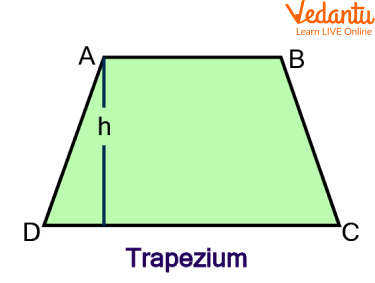Trapezium

As demonstrated in the diagram above, if a trapezium's height is "h," then

Trapezium perimeter = total lengths of all sides, which are AB + BC + CD + DA.

The area of the trapezium equals $\dfrac{1}{2} \times (AB + CD) \times h$

Q1. Find the base of a parallelogram, if its area is 144 square units and the height is 6 units.

Ans: Given, a parallelogram with;

Area = 144 square units

Height = 6 units

Therefore the area of the parallelogram = Base × Height

144 = Base $\times$ 6

Base = $\dfrac{114}{6}$ = 24 units

Q2. Calculate the quadrilateral's perimeter, which has sides of 2, 4, 9, and 15 cm.

Ans: We have the sides of a quadrilateral: 2 cm, 4 cm, 9 cm and 15 cm.

Therefore, the perimeter of a quadrilateral is given by the sum of all its sides, i.e.

P =  2 cm + 4 cm + 9 cm + 15 cm

P = 30 cm

Q 3. Find the missing angle x.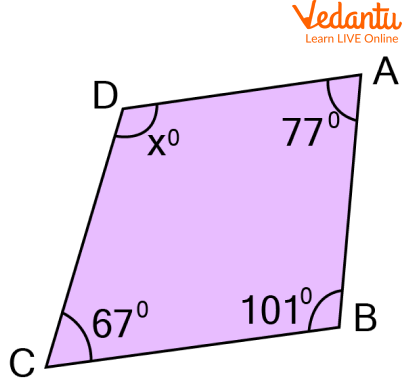Ans: We are aware that in a quadrilateral, the sum of all the angles is equal to $360^{\circ}$.

Therefore, we can express it as follows:

$x+77^{\circ}+101^{\circ}+67=360^{\circ}$

$x+245^{\circ}=360^{\circ}$

$x=360^{\circ}-245^{\circ}$

Hence, $x=115^{\circ}$.

Q1. The quadrilateral has three sides that measure 9 cm, 13 cm, and 17 cm in length, and its perimeter is 50 cm. Find the quadrilateral's missing side.

Ans: 11 cm.

Q 2. Find $\angle B C D$, if the figure at left, $\mathrm{ABCD}$ is a cyclic quadrilateral in which $\mathrm{AB} \| \mathrm{DC}$. If $\angle \mathrm{BAD}= 100^{\circ}$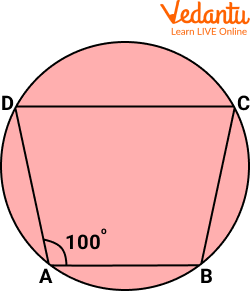Ans: $80^{\circ}$

## Summary

Let us summarise what we have learned throughout this article. A quadrilateral is a polygon with four sides and four angles, such as a square, parallelogram, rectangle, trapezium,  kite or rhombus. There are various types of quadrilaterals, depending on different characteristics, and shapes. The properties of quadrilaterals, namely square, parallelogram, rhombus, and trapezium are discussed, along with their solved examples, in brief in the above article. Try out the given practice questions to get a better understanding of the topic, quadrilateral.

## FAQs on Quadrilateral Practice Problems

1. Are all the quadrilaterals parallelograms?

No, all the quadrilaterals can never be parallelograms. For a quadrilateral to be a parallelogram, it must follow some conditions, such as the two sets of opposite sides must be parallel. Thus, we can say that every parallelogram is a quadrilateral but every quadrilateral need not be a parallelogram.

2. How do you calculate a quadrilateral's area?

The space occupied by a quadrilateral in two dimensions is referred to as the area of a quadrilateral. According to their characteristics, different kinds of quadrilaterals have different formulas for calculating area. For instance, the area of a square is equal to the square of its length, while a rectangle's area is equal to the product of length and width.